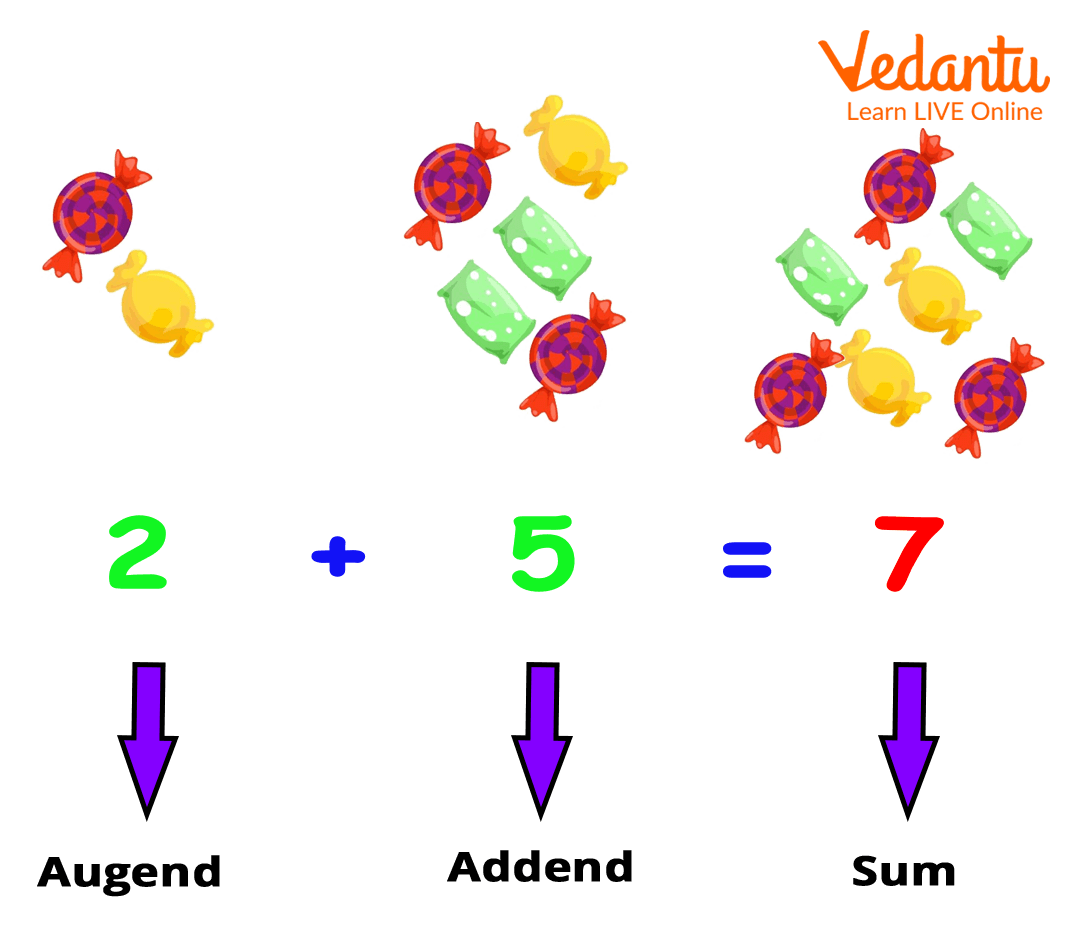Courses
Courses for Kids
Free study material
Free LIVE classes
More## Addend and Augend for Kids Mathematics

Piku has 5 chocolates. Kuku gives him 2 more chocolates. Now, how many chocolates does Piku have? Five plus two…..seven! Yes, you got it right. You get the answer by adding 5 and 2. Isn't it? Yes, ok. Then, do you know what 5 and 2 are called in mathematical terms?

Think…think my dear child….think.

They are called augend and addend, respectively. These are the components of addition. So, here we will know more about ‘augend’ and ‘addend’.

Addend is a number that is added to another number to make a sum. To understand this, we will consider an example. Suppose you went to the market with your little sister. You both buy two chocolates each and return home. When you enter the home, she is asking for one more chocolate. Now, being an elder brother or sister you gave one more chocolate to your little sister. The chocolate you gave her will be added. That is called ‘Addend’. Now your sister has three chocolates. Isn't it? Yes, Good Job!

2 chocolates + 1 chocolate = 3 chocolates

Where 2 chocolates are called augend and the 1 chocolate ‘to be’ added is called ‘addend’.

Now, let us understand it further with the help of some addends example:

Example 1: If you have 2 candies and your grandfather gives you 5 more, how many candies do you have?

Solution: So, to get the total candies, you need to add the candies which you had earlier and that your grandfather gave you. So, the sum would be 2 plus 5 equals 7.The pictorial representation of the given example is shown in the above image.

Example 2: Now, let us consider another example.

Solution: There is a dance performance in your school. The dance teacher told the monitor of your class that she needs 20 students. Your monitor has arranged 15 students so far. How many more students does your monitor need?

Since the monitor has arranged only 15 students, she needs 5 more students. The value ‘5’ is called an addend.

Now, we hope you understand what addend is. Here, we have some questions related to addition.

## Fill in the Blanks

1. 2 + ☐ = 8

2. 12 + 3 = ☐

3. ☐ + 20 = 25

4. 10 + ☐ = 20

5. 8 + 12 = ☐

The answers to the above-given questions are following:

1. 6 2. 15 3. 5 4. 10 5. 20

So, do you have the same answers?

If yes, then very good! If not, do not be sad. Just try again because practice makes a person perfect. We have several worksheets on our website for you to practise and master addition.

## Conclusion

Last updated date: 20th Sep 2023
Total views: 136.2k
Views today: 2.36k

## FAQs on What is Addend?

1. What is the difference between augend and addend?

Augend and addend are the numbers that are required to do addition. The number to which another number is added is called an augend, whereas the number which is added to an augend is called an addend.  Both the augends and addends are necessary. Also, if the addend is zero (0) then the sum will be the augend itself and if the augend is zero (0) then the sum will be ‘addend’.

2. What is an addend? Explain with an example.

Addend is a number that is to be added with another number.

Example: If you have five coins, and your father gives you two coins the next morning, then you have a total of 7 coins. So, here the additional two coins which are added are called the ‘addend’.